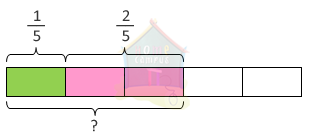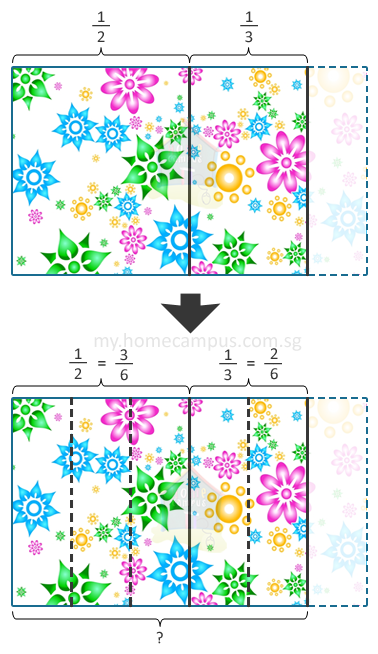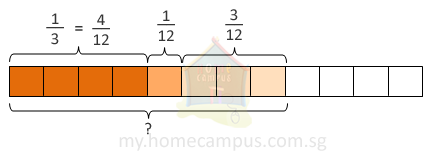Practice Unlimited Questions

Step 1: Express the fractions using a common denominator. For this you need to find the lowest common denominator.
Step 2: Create equivalent fractions by making the denominator common.
Step 3: Add the numerators and leave the denominator as is.
Step 4: Simplify fractions if required.

For better understanding follow the word problem below.

#### 1. Add 1 5 and 2 5 .1 5
+
 2 5

=
 1 + 2 5

=
 3 5

#### 2. Mrs. Singh had a cloth.      She cut 1 2 of the cloth for Cynthia and 1 3 of the cloth for Felicia.      What fraction of the cloth did she cut altogether for the two children?When the fractions to be added have different denominators, we must first change them to their equivalent fractions with the same denominator.

Cynthia:
 1 2
=
 3 6

Felicia:
 1 3
=
 2 6

Now, we add the two fractions as below:
 3 6
+
 2 6
=
 5 6

She cut
 5 6
of the cloth for the two children.

#### 3. Audrey had a melon.      She used 1 3 of it for a shake, 1 12 of it for a salad and 3 12 of it for an icecream.      What fraction of the melon did she use altogether?Shake:
 1 3
=
 4 12

 1 12

Icecream:
 3 12

 1 12
+
 1 12
+
 3 12

=
 4 12
+
 1 12
+
 3 12

=
 8 12

=
 2 3
(We must give our answer in the simplest form)

She used
 2 3
of the melon altogether.